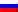# Bayesian optimization algorithm

Contenus

## Bayesian optimization algorithm

In a Bayesian optimization algorithm, the information processing objective is to build a probabilistic model that describes the relationships between the components of the fit solutions in the space of the problem. This is achieved by repeating the process of building and sampling from a Bayesian network that contains conditional dependencies, independencies, and conditional probabilities between the components of a solution.

The network is built from the relative frequencies of the components within a population of candidate high fitness solutions. Once the network is built, the candidate solutions are rejected and a new population of candidate solutions is generated from the model. The process is repeated until the model converges to a suitable prototype solution.

The following algorithm provides a pseudocode of the Bayesian optimization algorithm to minimize a cost function. The Bayesian network is built at each iteration using a greedy algorithm. The network is evaluated based on its correspondence with the information in the population of candidate solutions using either a Bayesian Dirichlet (BD) metric or a Bayesian Information Criterion (BIC).

The Bayesian optimization algorithm was designed and studied on basic binary string problems, most often representing binary function optimization problems.

Bayesian networks are typically built (expanded) from scratch with each iteration using an iterative process of adding, removing, and reversing links. In addition, earlier networks can be used as a basis for the next generation.

A greedy escalation algorithm is used at each algorithm iteration to optimize a Bayesian network to represent a population of candidate solutions.FRFRENRUES